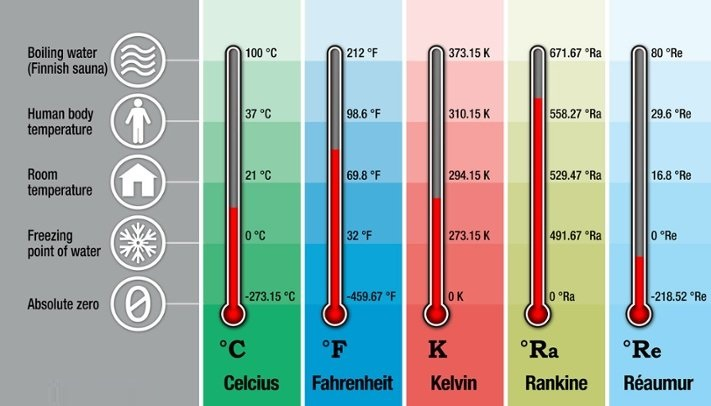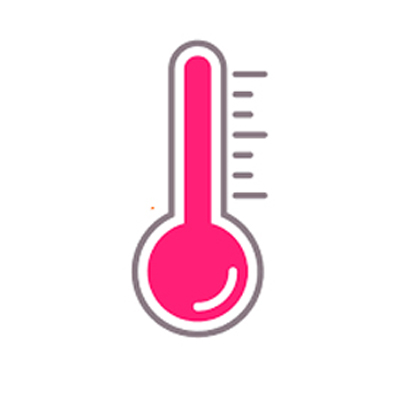# Convert Temperature Celsius, Fahrenheit, Kelvin, Rankine, Reaumur

An online tool to convert temperature in any scale like Celsius, Fahrenheit, Kelvin, Rankine, and Reaumur to another scale.

Steps: How to convert from one temperature scale to another

• First select the source temperature scale from the dropdown.
• Enter the value of source temperature.
• Click on the convert button to get temperatures in Celsius, Fahrenheit, Kelvin, Rankine, and Reaumur scale.

### Define Temperature

Temperature is the level of heat in a gas, liquid, or solid. Three scales are commonly used for measuring temperature. The Celsius and Fahrenheit scales are the most common. The Kelvin scale is primarily used in scientific experiments. The temperature of a substance is the degree of hotness or coldness of the substance. Or we can say, the temperature of a substance is an indication of the average kinetic energy of the molecules of the substance.

### Definition of Celsius Scale and its uses

The Celsius scale was invented in 1742 by the Swedish astronomer, Anders Celsius. This scale divides the range of temperature between the freezing and boiling temperatures of water into 100 equal parts. You will sometimes find this scale identified as the centigrade scale. Temperatures on the Celsius scale are known as degree Celsius (ºC).

Uses of Celsius scale: It is generally used for all scientific measurements.

#### Formula to convert temperature from Celsius to others

Fahrenheit = (C x 1.8) + 32

Kelvin = C + 273.15

Rankine = (C x 1.8) + 491.67

Reaumer = C x 0.8

### Definition of Fahrenheit Scale and its uses

The Fahrenheit scale was established by the German-Dutch physicist, Gabriel Daniel Fahrenheit, in 1724. While many countries now use the Celsius scale, the Fahrenheit scale is widely used in the United States. It divides the difference between the melting and boiling points of water into 180 equal intervals. Temperatures on the Fahrenheit scale are known as degree Fahrenheit (ºF).

Uses of Fahrenheit scale: It is generally used for engineering and houshold purposes.

#### Formula to convert temperature from Fahrenhiet to others

Celsius = (F - 32) / 1.8

Kelvin = (F + 459.67) / 1.8

Rankine = F + 459.67

Reaumer = (F - 32) / 2.25

### Definition of Kelvin Scale and its uses

The Kelvin scale is named after William Thompson Kelvin, a British physicist who devised it in 1848. It extends the Celsius scale down to absolute zero, a hypothetical temperature characterized by a complete absence of heat energy. Temperatures on this scale are called Kelvins (K).

Uses of Kelvin scale: The International System of Measurement uses kelvin scale for measuring temperature.

#### Formula to convert temperature from Kelvin to others

Celsius = K - 273.15

Fahrenhiet = (K x 1.8) - 459.67

Rankine = K x 1.8

Reaumer = (K - 273.15) * 0.8

### Definition of Rankine Scale and its uses

Rankine (ºRa) is named after William John Macquorn Rankine. Rankine cared about thermodynamics between times. The Rankine scale uses the smaller Fahrenheit degree for measurement - appropriate for a frugal Scot - and is another absolute temperature scale, as if we needed two of them.

Uses of Rankine scale: A few engineering fields in U.S. measures thermodynamic temperature using the Rankine scale.

#### Formula to convert temperature from Rankine to others

Celsius = (Ra - 32 - 459.67) / 1.8

Fahrenhiet = Ra - 459.67

Kelvin = Ra / 1.8

Reaumer = (Ra - 32 - 459.67) / 2.25

### Definition of Reaumur Scale and its uses

In Reaumur scale, freezing and boiling points of water are defined as 0 and 80 degrees respectively.

Uses of Reaumur scale: Its main modern uses are in some Italian and Swiss factories for measuring milk temperature during cheese production, and in the Netherlands for measuring temperature when cooking sugar syrup for desserts and sweets.

#### Formula to convert temperature from Reaumur to others

Celsius = Re x 1.25

Fahrenhiet = Re x 2.25 + 32

Kelvin = (Re x 1.25) + 273.15

Rankine = (Re x .25) + 32 + 459.67

#### Temperature Scale Table